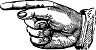PiPi, a mystical number some see as being “easy as pi.”And for others, it is only for those 'nerdy' peopleYet pi has found itself being useful, not only in geometry, (where it's all over the place) but also describing the motion of an object swinging on a string, in engineering applications, developing computer coding, studying properties of magnetism and the behavior of atoms.

But what is pi really? And where did it come from?

For starters, let's talk numbers. In particular, numbers that made sense to a Greek named Pythagoras. (He had a theorem some may recall).Pythagoras, and his followers, considered which numbers made sense to them. For starters, any counting number: 1, 2, 3, and so on. (The idea of zero came about a thousand years later.)

By the same token, you can divide numbers to get more numbers. In other words, fractions.These numbers, counting numbers and fractions, made sense to Pythagoras and those who came after him. Even to this day, we call these numbers (and zero as well) “rational numbers.”

At first, this sounds like a good idea. Consider the question of what number, multiplied by itself, equals 4.The answer, of course, is 2, 2 times 2 does equal 4. Since 2 is a counting number it is rational and everybody is happy.Now do the same problem again, but this time the numbers must multiply to 12.Houston, we now have a problem! To see why, go through the possible types of numbers we can use and still be rational. From counting numbers the closest we get is either 3, which is too small (3 times 3 is 9), or 4 which is too big (4 times 4 is 16). Trying to use simple fractions won't help either. So, like Goldilocks, we need something else that's just right.The just-right number cannot be rational. It is, in fact, called an "irrational number,” the special number here being 3.46410161513776 … Notice how the number keeps going on. This is a property of irrational numbers, their decimals go on forever without any apparent pattern.

I get around

Now consider how you can measure a circle. One way is straight across from one side to another, this is called the “diameter” (The prefix dia- means across or between). One can also measure the distance around the edge, called the “circumference.”How are the two related? Look at three circles.If you think about it, the smallest circle should have the smallest diameter ad circumference while the largest has the most. Also, if one again thinks about it, a circle that is two times as big as another should have two times the diameter and two times the circumference.

This can be expressed in a mathy way.The “some number” has to be the same for all circles of any size.

But what number? The ancient Greeks, Egyptians, and company knew it was something a little more than 3. For centuries afterwords, people refined the number, making it more accurate.

Now enter William Jones, a mathematician raised as a poor Welsh boy who grew to national prominence. (An interesting story) Mr. Jones, for the first time, called this special number pi (p) – the third letter in the Greek alphabet.

Now we have a familiar relationshipThe guest of honor

After Mr. Williams, it became clear that pi is, in fact, an irrational number. That is to say, the numbers after the decimal go on forever without forming any patterns.

The value of pi to the first several digits is:

3.141592653589793238462643383279502884197169

39937510582097...

If you want some serious digits of pi, there is a site that shows the first 100,000 digits. (Seriously!) The quest for more digits continues still. In fact, the known value of pi was recently extended to 31 trillion digits. The reason people pursue more digits to pi is not that the world needs a more accurate value – 31 trillion digits is more than anyone needs for their math calculations. Rather, this quest advances techniques to manage the massive amount of work involved in doing math with trillions of decimal places.

Pi trivia

The Guinness Book of World Records has the worst value of pi at 4; just plain old' 4. What? This doesn't even follow the rules of rounding! This was legislated by the Government of India, which is quite disappointing really, seeing as how India has produced several very capable mathematicians.

For so simple a basic idea and measurements of a circle, pi has taken on profound importance of its own. This journey to importance showcases our past struggle for growth, and our desire to learn more about the world around us, not just going around in circles.

Around the web

The Pi Song (Memorize 100 Digits Of π)

Don't miss out on future posts! Sign up for our email list and like us on Facebook!